# DAV Class 3 Maths Chapter 8 Worksheet 4 Solutions

The DAV Class 3 Maths Solutions and DAV Class 3 Maths Chapter 8 Worksheet 4 Solutions of Length offer comprehensive answers to textbook questions.

## DAV Class 3 Maths Ch 8 WS 4 Solutions

Question 1.
A shopkeeper had 35 m 50 cm cloth of one kind and 24 m 25 cm cloth of another kind. How much cloth did he have altogether?
Solution:
Length of I kind of cloth = 35 m 50 cm
Length of IInd kind of cloth = 24 m 25 cm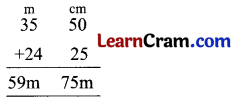Total length = 59 m 75 cm
He had 59 m 75 cm cloth altogether.

Question 2.
Rohan’s house is 8 km 250 m from school and Sohan’s house is 3 km 120 m from the school. Whose house is nearer to school and by how much?
Solution:
Sohan’s house is nearer to school
Distance of Rohan’s school = 8 km 250 m
Distance of Sohan’s school = 3 km 120 mAns = 5 km 130 mQuestion 3.
A roll of electric wire contains 85 m 75 cm of wire. If 46 m 55 cm of wire is used, how much wire is left in the roll?
Solution:
Wire in roll of electric wire = 85 m 75 cm
Wire used = 46 m 55 cm
Wire left = 39 m 20 cm39 m 20 cm wire is left in the roll.

Question 4.
John cycles 5 km 650 m in the morning and 8 km 300 m in the evening. How much distance does he cycle altogether?
Solution:
John cycles in morning = 5 km 650 m
John cycles in evening = 8 km 300 mCycle altogether = 13 km 950 cm
13 km 950 m cycle altogether.

Question 5.
Rita bought 30 m 70 cm cloth from a shop. She used 25 m 25 cm cloth for making curtains. How much cloth is left behind with her?
Solution:
Length of cloth shop = 30 m 70 cm
Cloth used for curtains = 25 m 25 cm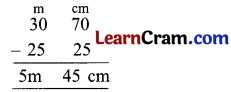Cloth left = 5 m 45 cm
5 m 45 cm cloth is left.### DAV Class 3 Maths Chapter 8 Value Based Questions

Rohan and Shyam are …………… gave it to Shyam.

Question 1.
How much cloth was left to make Shyam’s uniform.
Solution:
Total cloths she bought = 75 m 50 cm
She used for uniform = 72 m 25 cm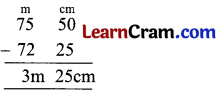Remaining cloth = 3 m 25 cm
3 m 25 m cloth was left for Shyam’s uniform.

Question 2.
In what other ways can Rohan be helpful to Shyam?
Solution:
(a) By giving him stationary materials, like pencil box, pen, notebook as a gift.
(b) By giving him school bag stitched by (Rohan’s) mother.

Question 3.
What do you think about Rohan and his mother’s nature.
Solution:
They are kind by nature.Question 1.
Change into centimetres.

(a) 0.35 m
Solution:
0.35 m = 0.35 × 100
(1 m = 100 cm)
= 35 cm

(b) 4.50 m
Solution:
4.50 m = 4.50 × 100 cm
(1 m = 100 cm)
= 450 cm

(c) 7 m
Solution:
7 m = 7 × 100 cm
(1 m = 100 cm)
= 700 cmQuestion 2.
Change into metres and centimetres.

(a) 1342 cm
Solution:
1342 cm (Divide by 100)
1342 ÷ 100
= 13.42 m
= 13 m 42 cm.

(b) 2017 cm
Solution:
2017 cm = 2017 ÷ 100
= 20.17 m
= 20 m 17 cm.

(c) 318 cm
Solution:
318 cm = 318 ÷ 100 m
= 3.18 m
= 3 m 18 cm.Question 3.
Change in kilometre.

(a) 3012 m
Solution:
3012 ÷ 1000 km
(1 kilometre = 1000 m)
= 3.012 km

(b) 2589 m
Solution:
2589 ÷ 1000 km
= 2.589 km

(c) 5600 m
Solution:
5600 ÷ 1000 km
= 5.600 kmQuestion 4.
Change into metres.

(a) 6 km
Solution:
6 km (1 km = 1000 m)
= 6 × 1000 m
= 6000 m

(b) 90 km
Solution:
90 km (1 km = 1000 m)
= 90 × 1000 m
= 90000 m

(c) 82 km
Solution:
82 km (1 km = 1000 m)
= 82 × 1000 m
= 82000 mQuestion 5.

(a) 42 km 369 m and 17 km 441 m
Solution: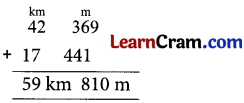(b) 432 km 574 m and 60 km, 103 m and 60 m.
Solution:Question 6.
A carpet is 13 m 10 cm long. Joya cut the carpet 5 m 20 cm according to her use in bedroom. What is remaining length of carpet?
Solution:
Length of carpet = 13 m 10 cm
Length of carpet cut = 5 m 20 cm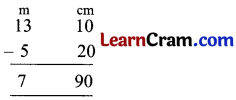Remaining carpet = 7 m 90 cm
7 m 90 cm carpet remained.### DAV Class 3 Maths Chapter 8 Worksheet 4 Notes

Word Problems

Question 1.
Manu has a rope in small pieces. He has rope of length 9 m 20cm, 10m 36 cm and 20 m 25 cm. Find the total length of rope.
Solution:
Length of I piece = 9 m 20 cm
Length of IInd piece = 10 m 36 cm
Length of IIIrd piece = 20 m 25 cm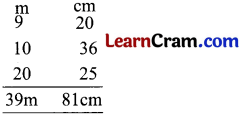Total length = 39 m 81 cmQuestion 2.
Ramesh had to travel 15 km 370 m to reach hospital. Ramesh travelled 10 km by auto. What distance has he to travel now?
Solution:
Distance to hospital = 15 km 370 m
Distance travelled by auto = 10 km 000 m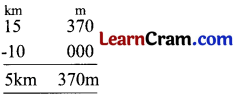Remaining distance = 5km 370m
Ramesh has yet to travel 5 km 370 m.